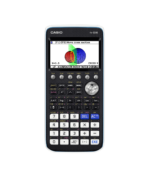Teaching Trigonometry Archives - Casio Calculators

# Teaching Trigonometry

Displaying Resources

FilterTeach Leaflet
fx-CG50 A-Level IB
Derivative of Trigonometric Functions

The investigation looks at the gradient of tangents to each of the basic trigono...Teach Leaflet
fx-CG50 IB A-Level
Harmonic Form in Trigonometry

This investigation explores the shapes of graphs of the form y = acos x + bsin x...Teach Leaflet
fx-CG50 A-Level IB
Inverse Trigonometric Functions

This investigation allows learners to draw and compare the graphs of y = cos x, ...Teach Leaflet
fx-CG50 A-Level IB
Reciprocal Trigonometric Functions

This activity guides learners to sketch the reciprocal trigonometric graphs onto...Teach Leaflet
fx-CG50 A-Level IB
Combined Transformation of Trig Graphs

This guided investigation uses the calculator functionality to transform a graph...Teach Leaflet
fx-CG50 A-Level GCSE IB
Single Transformation of Trig Graphs

This activity requires learners to match the equation of a transformed trigonome...Teach Leaflet
fx-CG50 A-Level IB
Trigonometric Equations Using Identities

The activity provides practice in sorting trigonometric equations where more tha...Teach Leaflet
fx-CG50 A-Level GCSE IB
Basic Trigonometric Equations

This is an introductory matching exercise focusing on finding two solutions of t...